cancel
Showing results for
Did you mean:
See all
See all
##### Top groups
Explore all groups

# It's not the same without you

Join the community to find out what other Atlassian users are discussing, debating and creating.# Conditional Formatting for Confluence tables

I want to format a column in my table so that the dates passing today would turn red and if the date is closer than or equal to 6 months from now it would turn yellow. I'm not too sure about how to do this on confluence. If there's a method you can help me out with, I'd really appreciate it. Thank you!

#### 1 accepted

You may try the Table Filter and Charts for Confluence app and its Table Transformer macro with the advanced cells formatting feature.

Wrap your table in the Table Transformer macro: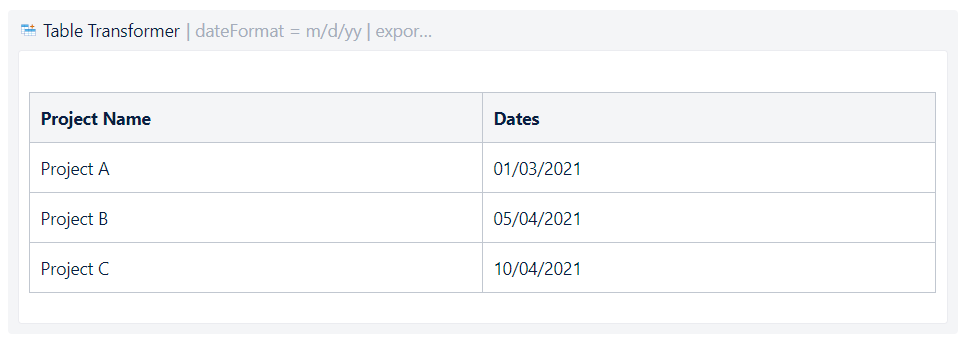Set an appropriate date format: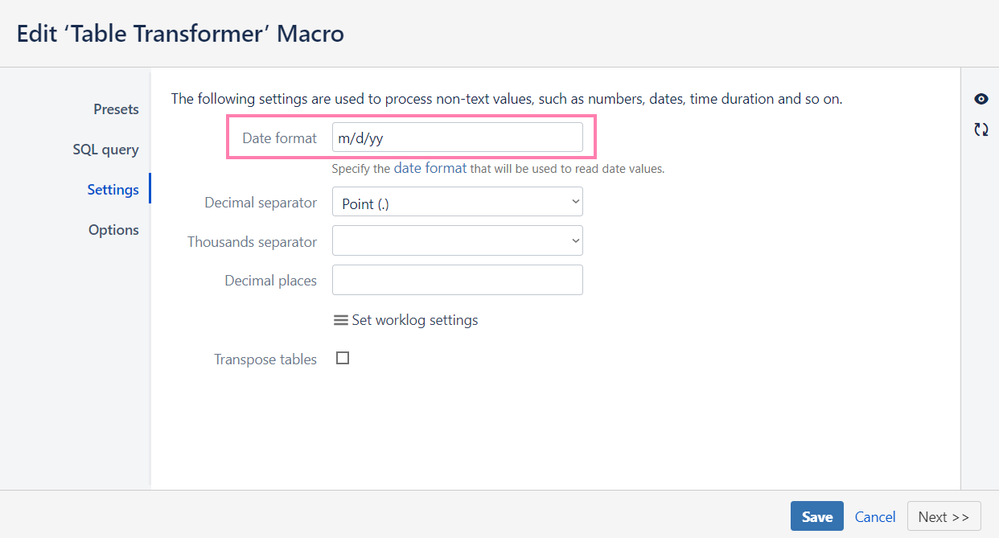And use the following custom SQL query to count the period between two dates and format your cells:

SELECT T1.'Project Name',
FORMATWIKI("{cell:bgColor=" +
CASE WHEN DATEDIFF(day,"today",'Dates') <= "0" THEN "#FA7E70"
WHEN DATEDIFF(day,"today",'Dates') > "0" AND DATEDIFF(day,"today",'Dates') <= "180" THEN "#FFE784"
ELSE "#8FCA7D"
END
+ "}" + T1.'Dates' + "{cell}")
AS 'Dates'
FROM T1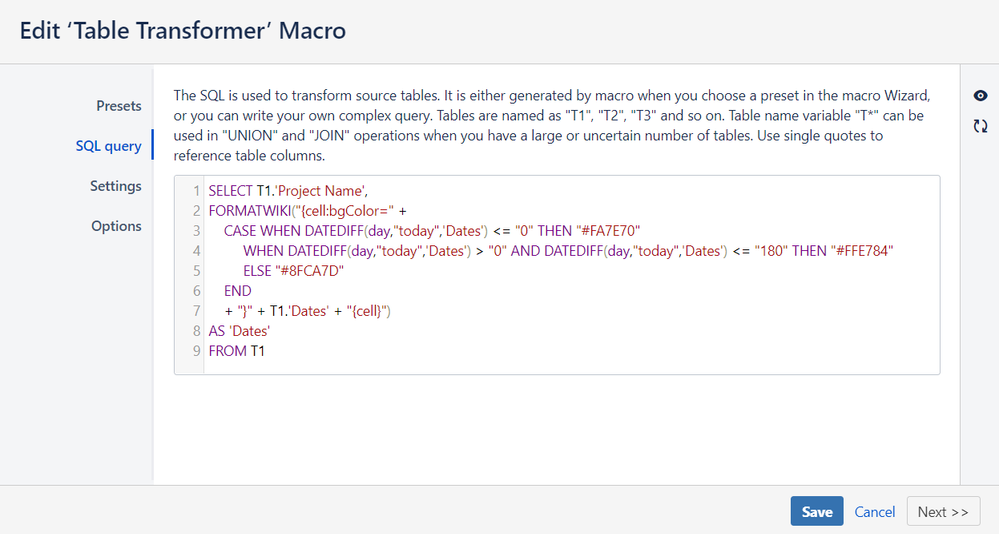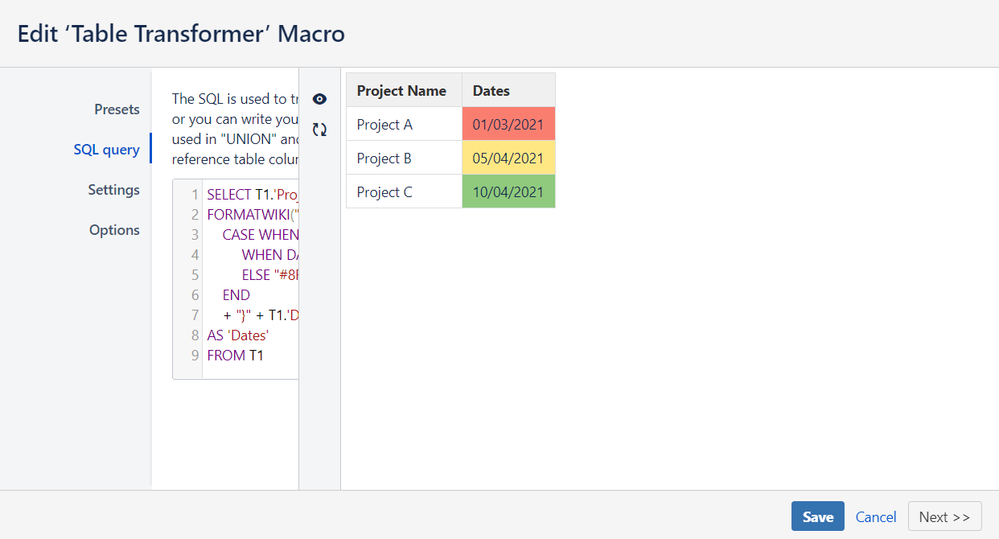Hope this may help your case.

Hello there,

Thank you for your response, I have a quick question. Why have you mentioned 'Project Name' after selecting T1? In my case should I write 'Certification' ?

Also I have multiple tables like this that needs the same formatting, does that mean I should type in 'T*' instead of specifying the table?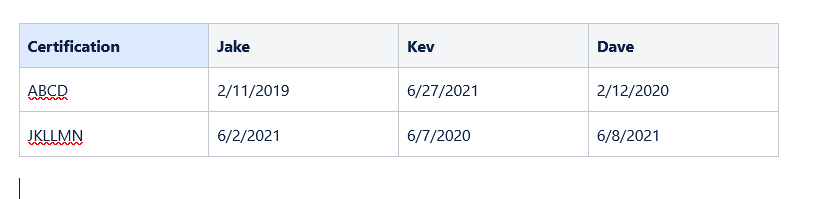If you want all the columns from your table to be displayed, than use an asterisk:

SELECT *,
FORMATWIKI ...

If you want to show only specific columns, than list them:

SELECT T1.'Certification', T1.'Jake' ...

In the end of your SQL query you can type T1 or T* - it makes no difference because you have only one table inside your Table Transformer macro.

But you need to use a specific column name while applying conditional formatting ('Dates' for my case and, for example, 'Kev' for yours).

Hello there, since I have more than one column that needs conditional formatting, should I separate it with a comma like this

CASE WHEN DATEDIFF(day,"today",Jake', 'Kev', 'Dave') <= "0" THEN "#FA7E70"

Or should I rewrite the code for every column?

Also I keep getting an output like this for some reason, it doesn't display the date, but some random numbers. Can you tell me why?

Thank you!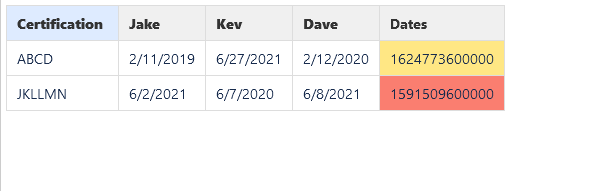For the first question: you should apply conditional formatting separately for every required column ('Jake', 'Kev' and 'Dave'). You compare every column date with "today" so you can't list several columns at once via commas.

SELECT *,
FORMATWIKI("{cell:bgColor=" +
CASE WHEN DATEDIFF(day,"today",'Jake') <= "0" THEN "#FA7E70"
WHEN DATEDIFF(day,"today",'Jake') > "0" AND DATEDIFF(day,"today",'Jake') <= "180" THEN "#FFE784"
ELSE "#8FCA7D"
END
+ "}" + T1.'Jake' + "{cell}")
AS 'Jake',
FORMATWIKI("{cell:bgColor=" +
CASE WHEN DATEDIFF(day,"today",'Kev') <= "0" THEN "#FA7E70"
WHEN DATEDIFF(day,"today",'Kev') > "0" AND DATEDIFF(day,"today",'Kev') <= "180" THEN "#FFE784"
ELSE "#8FCA7D"
END
+ "}" + T1.'Kev' + "{cell}")
AS 'Kev',
FORMATWIKI("{cell:bgColor=" +
CASE WHEN DATEDIFF(day,"today",'Dave') <= "0" THEN "#FA7E70"
WHEN DATEDIFF(day,"today",'Dave') > "0" AND DATEDIFF(day,"today",'Dave') <= "180" THEN "#FFE784"
ELSE "#8FCA7D"
END
+ "}" + T1.'Dave' + "{cell}")
AS 'Dave'
FROM T*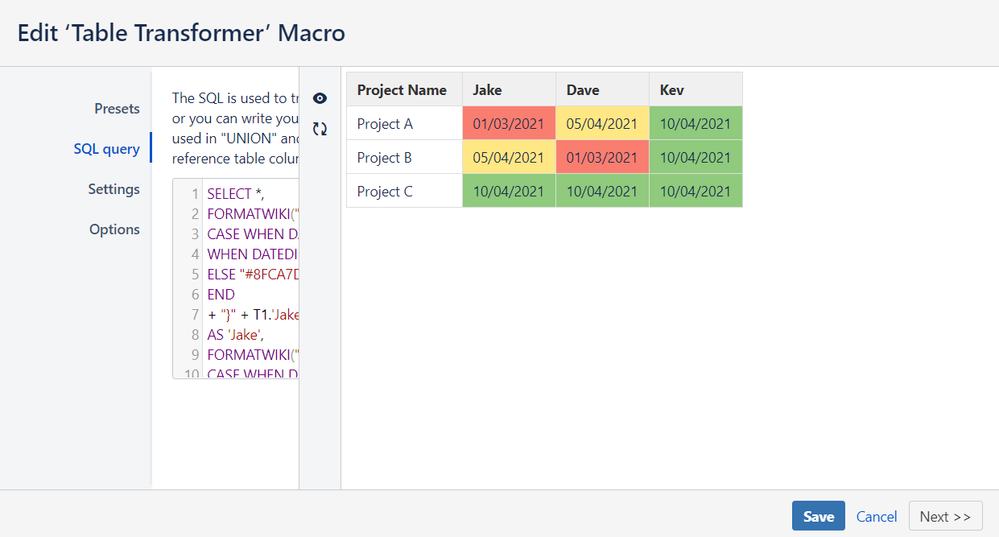For the second question: make sure that your date format corresponds the fornat inside the Table Transformer macro (I've shown it in my very first answer).

And you shouldn't have the 'Dates' column at all - it was from my example, so you didn't change the SQL query. Now I've given the whole SQL query for you table, so you may just copy it.

@Katerina Kovriga _Stiltsoft_  I seems to have come across a problem with the table when I published it.

Can you tell me why. Thanks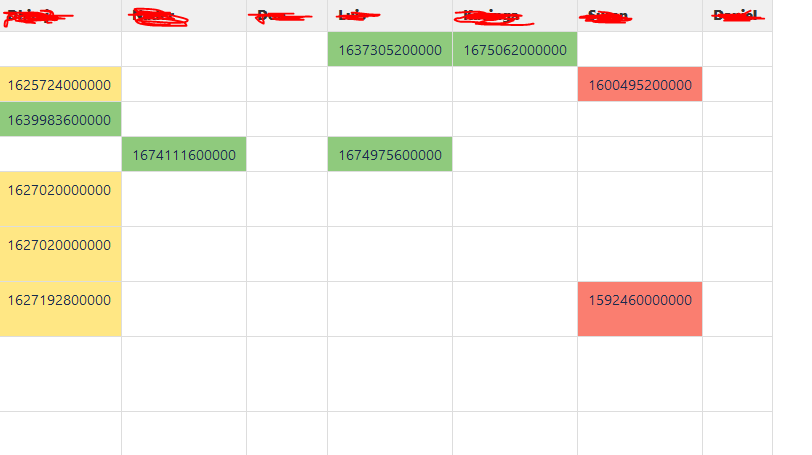Hi, please, check again your SQL query (you should compare every column with "today" separately) and the date format in the Table Transformer macro (Settings -> Date format, it should correspond the date format inside your original table).

To find a mistake, we need to see screenshots of your original table and the Settings tab of the Table Transformer macro along with a copy of your SQL query.

Maybe it will be better to raise a support ticket and attach everything there not to show any confidential data in the Community.

I've attached the screenshots and the code below.

SELECT *,
FORMATWIKI("{cell:bgColor=" +
CASE WHEN DATEDIFF(day,"today",'Jack') <= "0" THEN "#FA7E70"
WHEN DATEDIFF(day,"today",'Jack') > "0" AND DATEDIFF(day,"today",'Jack') <= "180" THEN "#FFE784"
ELSE "#8FCA7D"
END
+ "}" + T1.'Jack' + "{cell}")
AS 'Jack',
FORMATWIKI("{cell:bgColor=" +
CASE WHEN DATEDIFF(day,"today",'Nick') <= "0" THEN "#FA7E70"
WHEN DATEDIFF(day,"today",'Nick') > "0" AND DATEDIFF(day,"today",'Nick') <= "180" THEN "#FFE784"
ELSE "#8FCA7D"
END
+ "}" + T1.'Nick' + "{cell}")
AS 'Nick',
FORMATWIKI("{cell:bgColor=" +
CASE WHEN DATEDIFF(day,"today",'Jake') <= "0" THEN "#FA7E70"
WHEN DATEDIFF(day,"today",'Jake') > "0" AND DATEDIFF(day,"today",'Jake') <= "180" THEN "#FFE784"
ELSE "#8FCA7D"
END
+ "}" + T1.'Jake' + "{cell}")
AS 'Jake',
FORMATWIKI("{cell:bgColor=" +
CASE WHEN DATEDIFF(day,"today",'Kev') <= "0" THEN "#FA7E70"
WHEN DATEDIFF(day,"today",'Kev') > "0" AND DATEDIFF(day,"today",'Kev') <= "180" THEN "#FFE784"
ELSE "#8FCA7D"
END
+ "}" + T1.'Kev' + "{cell}")
AS 'Kev',
FORMATWIKI("{cell:bgColor=" +
CASE WHEN DATEDIFF(day,"today",'Nisal') <= "0" THEN "#FA7E70"
WHEN DATEDIFF(day,"today",'Nisal') > "0" AND DATEDIFF(day,"today",'Nisal') <= "180" THEN "#FFE784"
ELSE "#8FCA7D"
END
+ "}" + T1.'Nisal' + "{cell}")
AS 'Nisal',
FORMATWIKI("{cell:bgColor=" +
CASE WHEN DATEDIFF(day,"today",'Paul') <= "0" THEN "#FA7E70"
WHEN DATEDIFF(day,"today",'Paul') > "0" AND DATEDIFF(day,"today",'Paul') <= "180" THEN "#FFE784"
ELSE "#8FCA7D"
END
+ "}" + T1.'Paul' + "{cell}")
AS 'Paul',
FORMATWIKI("{cell:bgColor=" +
CASE WHEN DATEDIFF(day,"today",'Dave') <= "0" THEN "#FA7E70"
WHEN DATEDIFF(day,"today",'Dave') > "0" AND DATEDIFF(day,"today",'Dave') <= "180" THEN "#FFE784"
ELSE "#8FCA7D"
END
+ "}" + T1.'Dave' + "{cell}")
AS 'Dave'
FROM T*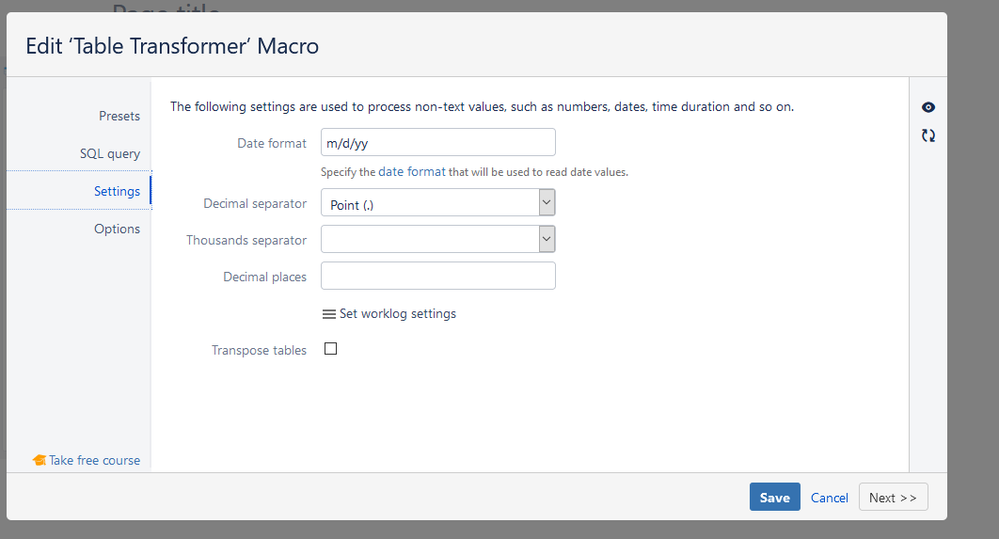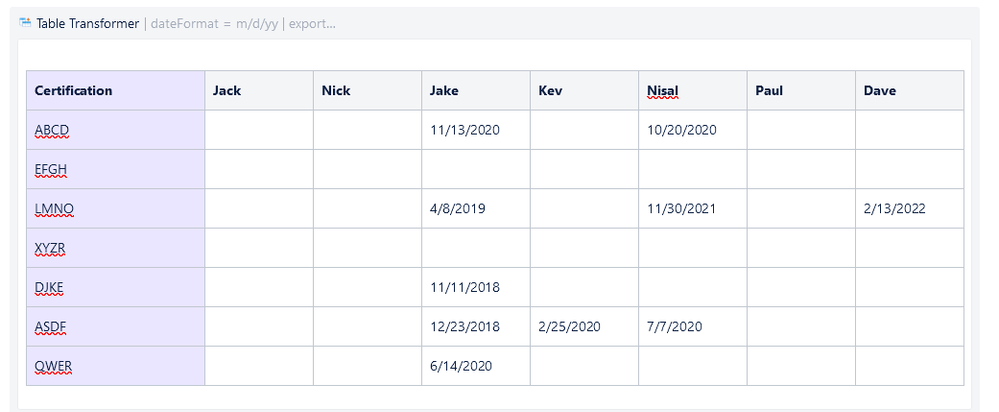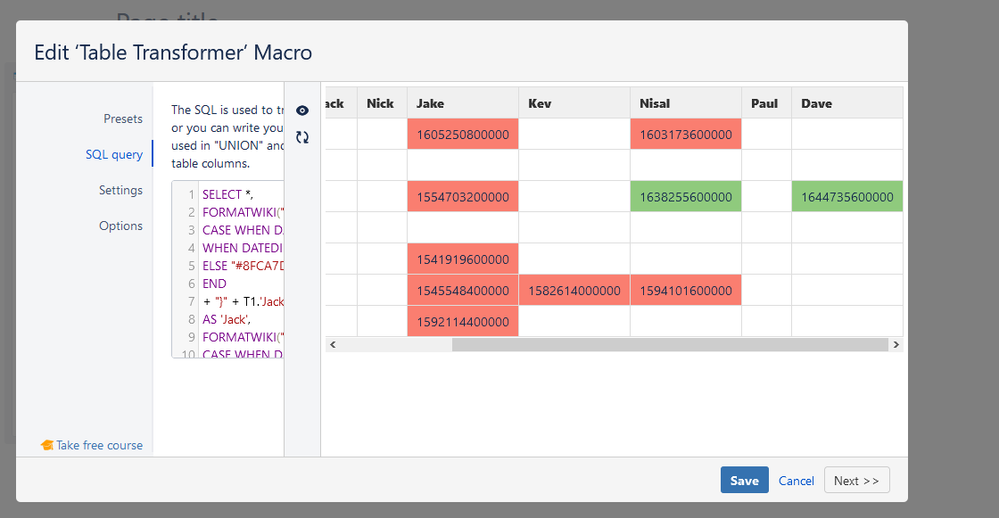I think there's a problem with the app because I edited the way I entered the date. Instead of 2/25/2020 under Kev I entered 02/25/2020, and it seems the problem is fixed but this isn't the case if I want to enter a date in November (eg: 11/11/2018)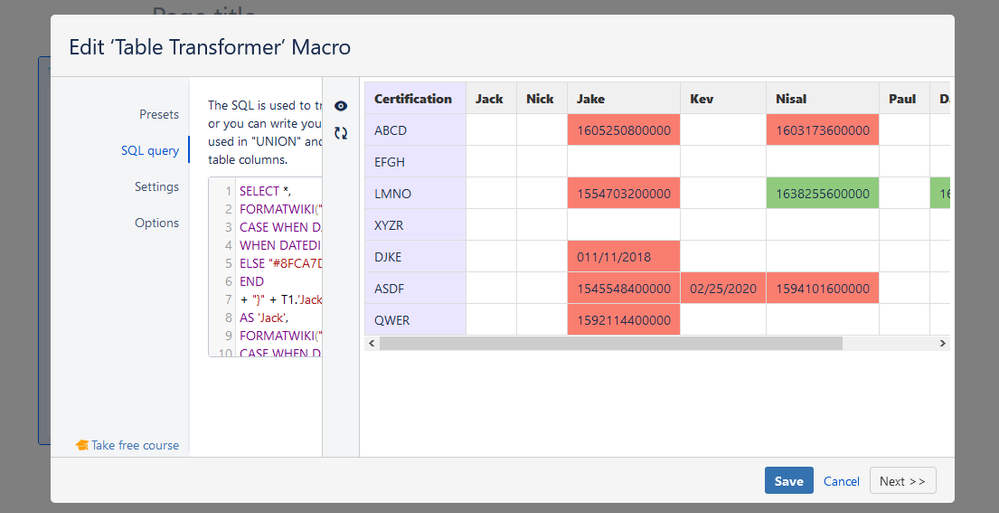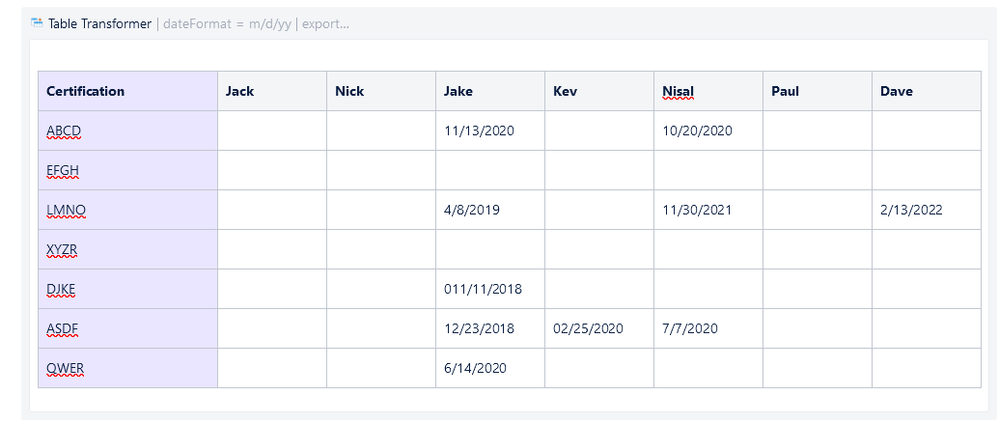@Pansilu Diegayu Wickramasinghe , sorry it's my bad: forgot about the FORMATDATE expression in the SQL query.

FORMATWIKI("{cell:bgColor=" +
CASE WHEN DATEDIFF(day,"today",'Jack') <= "0" THEN "#FA7E70"
WHEN DATEDIFF(day,"today",'Jack') > "0" AND DATEDIFF(day,"today",'Jack') <= "180" THEN "#FFE784"
ELSE "#8FCA7D"
END
+ "}" + FORMATDATE(T1.'Jack') + "{cell}")
AS 'Jack'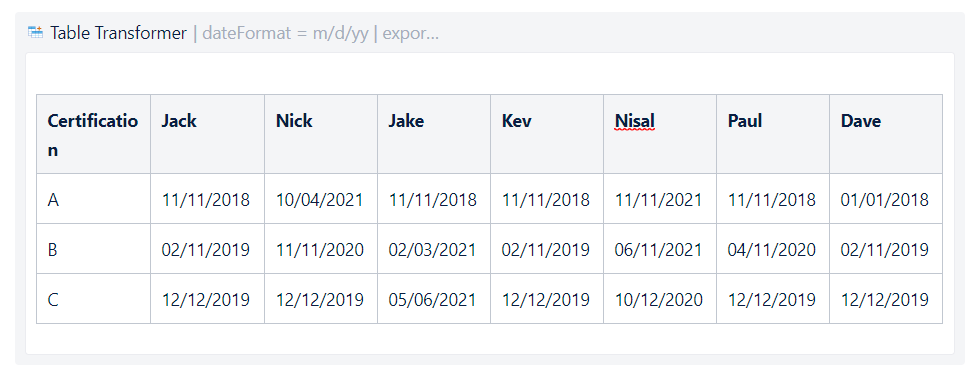SELECT *,
FORMATWIKI("{cell:bgColor=" +
CASE WHEN DATEDIFF(day,"today",'Jack') <= "0" THEN "#FA7E70"
WHEN DATEDIFF(day,"today",'Jack') > "0" AND DATEDIFF(day,"today",'Jack') <= "180" THEN "#FFE784"
ELSE "#8FCA7D"
END
+ "}" + FORMATDATE(T1.'Jack') + "{cell}")
AS 'Jack',
FORMATWIKI("{cell:bgColor=" +
CASE WHEN DATEDIFF(day,"today",'Nick') <= "0" THEN "#FA7E70"
WHEN DATEDIFF(day,"today",'Nick') > "0" AND DATEDIFF(day,"today",'Nick') <= "180" THEN "#FFE784"
ELSE "#8FCA7D"
END
+ "}" + FORMATDATE(T1.'Nick') + "{cell}")
AS 'Nick',
FORMATWIKI("{cell:bgColor=" +
CASE WHEN DATEDIFF(day,"today",'Jake') <= "0" THEN "#FA7E70"
WHEN DATEDIFF(day,"today",'Jake') > "0" AND DATEDIFF(day,"today",'Jake') <= "180" THEN "#FFE784"
ELSE "#8FCA7D"
END
+ "}" + FORMATDATE(T1.'Jake') + "{cell}")
AS 'Jake',
FORMATWIKI("{cell:bgColor=" +
CASE WHEN DATEDIFF(day,"today",'Kev') <= "0" THEN "#FA7E70"
WHEN DATEDIFF(day,"today",'Kev') > "0" AND DATEDIFF(day,"today",'Kev') <= "180" THEN "#FFE784"
ELSE "#8FCA7D"
END
+ "}" + FORMATDATE(T1.'Kev') + "{cell}")
AS 'Kev',
FORMATWIKI("{cell:bgColor=" +
CASE WHEN DATEDIFF(day,"today",'Nisal') <= "0" THEN "#FA7E70"
WHEN DATEDIFF(day,"today",'Nisal') > "0" AND DATEDIFF(day,"today",'Nisal') <= "180" THEN "#FFE784"
ELSE "#8FCA7D"
END
+ "}" + FORMATDATE(T1.'Nisal') + "{cell}")
AS 'Nisal',
FORMATWIKI("{cell:bgColor=" +
CASE WHEN DATEDIFF(day,"today",'Paul') <= "0" THEN "#FA7E70"
WHEN DATEDIFF(day,"today",'Paul') > "0" AND DATEDIFF(day,"today",'Paul') <= "180" THEN "#FFE784"
ELSE "#8FCA7D"
END
+ "}" + FORMATDATE(T1.'Paul') + "{cell}")
AS 'Paul',
FORMATWIKI("{cell:bgColor=" +
CASE WHEN DATEDIFF(day,"today",'Dave') <= "0" THEN "#FA7E70"
WHEN DATEDIFF(day,"today",'Dave') > "0" AND DATEDIFF(day,"today",'Dave') <= "180" THEN "#FFE784"
ELSE "#8FCA7D"
END
+ "}" + FORMATDATE(T1.'Dave') + "{cell}")
AS 'Dave'
FROM T*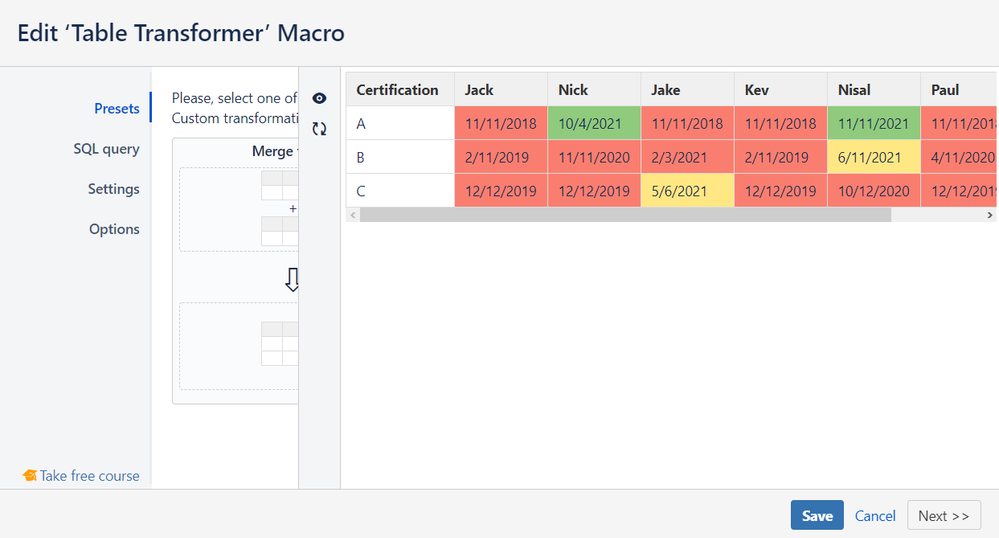Hello @Katerina Kovriga _Stiltsoft_  this made all my empty cells red as well.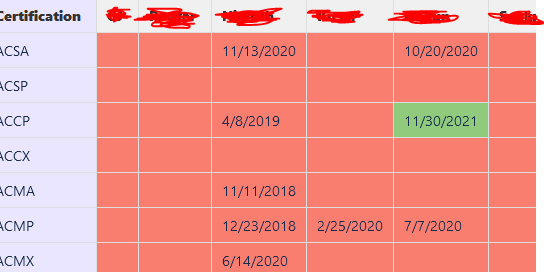If you have empty cells in your table and want them to be white (or any other color you wish), add an additional "when" entry into your query:

WHEN T1.'Jack' IS NULL THEN "#FFFFFF"

Here is an example for T1.'Jack' with this additional entry and T1.'Nick' without it:

SELECT *,
FORMATWIKI("{cell:bgColor=" +
CASE
WHEN T1.'Jack' IS NULL THEN "#FFFFFF"
WHEN DATEDIFF(day,"today",'Jack') <= "0" THEN "#FA7E70"
WHEN DATEDIFF(day,"today",'Jack') > "0" AND DATEDIFF(day,"today",'Jack') <= "180" THEN "#FFE784"
ELSE "#8FCA7D"
END
+ "}" + FORMATDATE(T1.'Jack') + "{cell}")
AS 'Jack',
FORMATWIKI("{cell:bgColor=" +
CASE WHEN DATEDIFF(day,"today",'Nick') <= "0" THEN "#FA7E70"
WHEN DATEDIFF(day,"today",'Nick') > "0" AND DATEDIFF(day,"today",'Nick') <= "180" THEN "#FFE784"
ELSE "#8FCA7D"
END
+ "}" + FORMATDATE(T1.'Nick') + "{cell}")
AS 'Nick'
FROM T*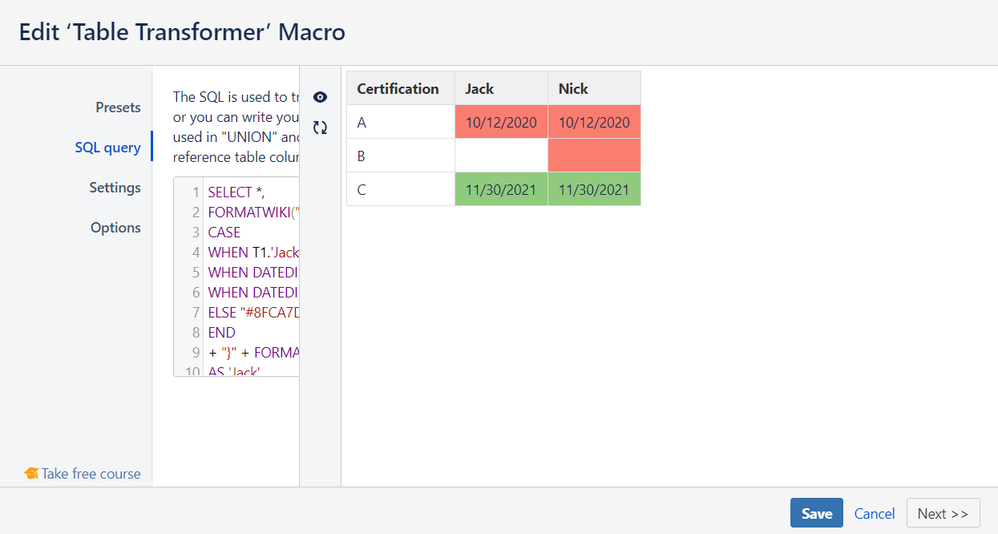Hi @Katerina Kovriga _Stiltsoft_  this worked. I have one last question. I have cells with a text such as " No expiration" and I want these cells to be green. How would I write a code for this?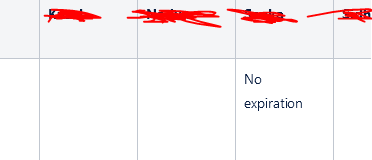Try the following query:

SELECT *,
FORMATWIKI("{cell:bgColor=" +
CASE
WHEN T1.'Jack' IS NULL THEN "#FFFFFF"
WHEN DATEDIFF(day,"today",'Jack') <= "0" THEN "#FA7E70"
WHEN DATEDIFF(day,"today",'Jack') > "0" AND DATEDIFF(day,"today",'Jack') <= "180" THEN "#FFE784"
ELSE "#8FCA7D"
END
+ "}" + IF(T1.'Jack' > 0, FORMATDATE(T1.'Jack'), T1.'Jack') + "{cell}")
AS 'Jack'
FROM T*

@Katerina Kovriga _Stiltsoft_
Hi there, i found this Q/A very helpfull, but i am stuck on 2 things.

I am using your above examples to shade the bg of the cells in my table based on current day == current week.

I cant however get the cell that is NULL to shade (it works when its not null).

Also I wanted to add another CASE so that any cell with a preceding '*" would have the text in cell turned red?  any help would be great!  thanks in advance.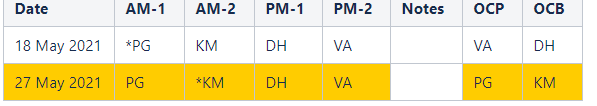Here is my current SQL

SELECT *,
FORMATWIKI("{cell:bgColor=" +
CASE WHEN DATEDIFF(day,"today",'Date') <= "7" AND DATEDIFF(day,"today",'Date') >= "0" THEN "#FFCC00"
ELSE "#FFFFFF"
END
+ "}" + FORMATDATE(T1.'Date') + "{cell}")
AS 'Date',
FORMATWIKI("{cell:bgColor=" +
CASE WHEN DATEDIFF(day,"today",'Date') <= "7" AND DATEDIFF(day,"today",'Date') >= "0" THEN "#FFCC00"
ELSE "#FFFFFF"
END
+ "}" + T1.'AM-1' + "{cell}")
AS 'AM-1',
FORMATWIKI("{cell:bgColor=" +
CASE WHEN DATEDIFF(day,"today",'Date') <= "7" AND DATEDIFF(day,"today",'Date') >= "0" THEN "#FFCC00"
ELSE "#FFFFFF"
END
+ "}" + T1.'AM-2' + "{cell}")
AS 'AM-2',
FORMATWIKI("{cell:bgColor=" +
CASE WHEN DATEDIFF(day,"today",'Date') <= "7" AND DATEDIFF(day,"today",'Date') >= "0" THEN "#FFCC00"
ELSE "#FFFFFF"
END
+ "}" + T1.'PM-1' + "{cell}")
AS 'PM-1',
FORMATWIKI("{cell:bgColor=" +
CASE WHEN DATEDIFF(day,"today",'Date') <= "7" AND DATEDIFF(day,"today",'Date') >= "0" THEN "#FFCC00"
ELSE "#FFFFFF"
END
+ "}" + T1.'PM-2' + "{cell}")
AS 'PM-2',
## this is the null field.
FORMATWIKI("{cell:bgColor=" +

CASE WHEN DATEDIFF(day,"today",'Date') <= "7" AND DATEDIFF(day,"today",'Date') >= "0" THEN "#FFCC00"
ELSE "#FFFFFF"
END
+ "}" + T1.'Notes' + "{cell}")
AS 'Notes',
FORMATWIKI("{cell:bgColor=" +
CASE WHEN DATEDIFF(day,"today",'Date') <= "7" AND DATEDIFF(day,"today",'Date') >= "0" THEN "#FFCC00"
ELSE "#FFFFFF"
END
+ "}" + T1.'OCP' + "{cell}")
AS 'OCP',
FORMATWIKI("{cell:bgColor=" +
CASE WHEN DATEDIFF(day,"today",'Date') <= "7" AND DATEDIFF(day,"today",'Date') >= "0" THEN "#FFCC00"
ELSE "#FFFFFF"
END
+ "}" + T1.'OCB' + "{cell}")
AS 'OCB'

FROM T*

Hi @Paul Gwin ,

Wrap your table into the two Table Transformer macros subsequently (if your hosting is Cloud, then use the Table Toolbox to nest several macros):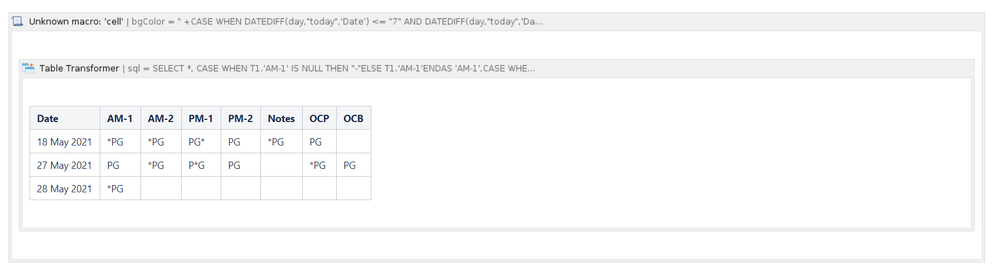Use the following SQL for the first (internal) Transformer:

SELECT *,
CASE WHEN T1.'AM-1' IS NULL THEN "-"
ELSE T1.'AM-1'
END
AS 'AM-1',
CASE WHEN T1.'AM-2' IS NULL THEN "-"
ELSE T1.'AM-2'
END
AS 'AM-2',
CASE WHEN T1.'PM-1' IS NULL THEN "-"
ELSE T1.'PM-1'
END
AS 'PM-1',
CASE WHEN T1.'PM-2' IS NULL THEN "-"
ELSE T1.'PM-2'
END
AS 'PM-2',
CASE WHEN T1.'Notes' IS NULL THEN "-"
ELSE T1.'Notes'
END
AS 'Notes',
CASE WHEN T1.'OCP' IS NULL THEN "-"
ELSE T1.'OCP'
END
AS 'OCP',
CASE WHEN T1.'OCB' IS NULL THEN "-"
ELSE T1.'OCB'
END
AS 'OCB'
FROM T*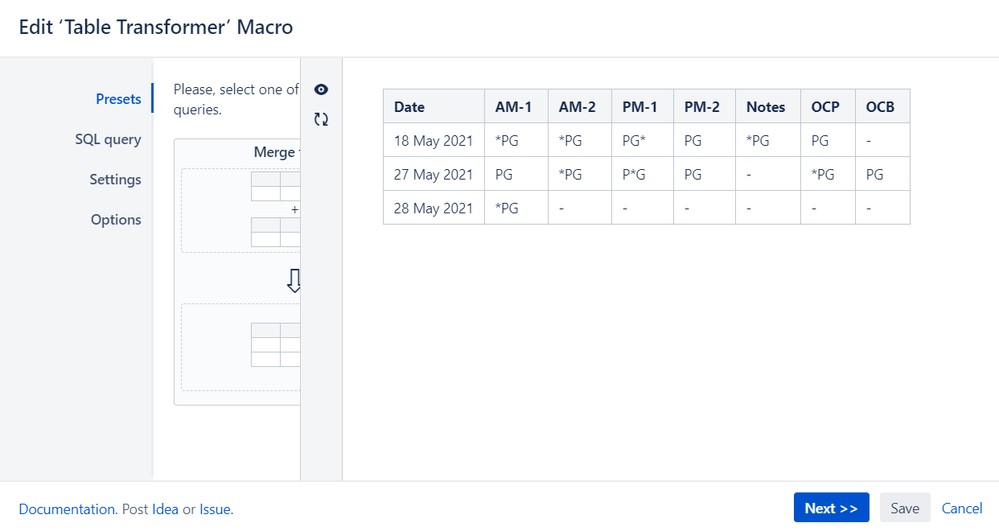Now your empty cells are not empty and you'll be able to color the background.

And here is the SQL for your second Transformer:

SELECT *,
FORMATWIKI("{cell:bgColor=" +
CASE WHEN DATEDIFF(day,"today",'Date') <= "7" AND DATEDIFF(day,"today",'Date') >= "0" THEN "#FFCC00"
ELSE "#FFFFFF"
END
+ "}" + FORMATDATE(T1.'Date') + "{cell}")
AS 'Date',
FORMATWIKI("{cell:bgColor=" +
CASE WHEN DATEDIFF(day,"today",'Date') <= "7" AND DATEDIFF(day,"today",'Date') >= "0" AND T1.'AM-1' NOT LIKE "*%" THEN "#FFCC00"
WHEN DATEDIFF(day,"today",'Date') <= "7" AND DATEDIFF(day,"today",'Date') >= "0" AND T1.'AM-1' LIKE "*%" THEN "RED"
WHEN T1.'AM-1' LIKE "*%" THEN "RED"
ELSE "#FFFFFF"
END
+ "}" + T1.'AM-1' + "{cell}")
AS 'AM-1',
FORMATWIKI("{cell:bgColor=" +
CASE WHEN DATEDIFF(day,"today",'Date') <= "7" AND DATEDIFF(day,"today",'Date') >= "0" AND T1.'AM-2' NOT LIKE "*%" THEN "#FFCC00"
WHEN DATEDIFF(day,"today",'Date') <= "7" AND DATEDIFF(day,"today",'Date') >= "0" AND T1.'AM-2' LIKE "*%" THEN "RED"
WHEN T1.'AM-2' LIKE "*%" THEN "RED"
ELSE "#FFFFFF"
END
+ "}" + T1.'AM-2' + "{cell}")
AS 'AM-2',
FORMATWIKI("{cell:bgColor=" +
CASE WHEN DATEDIFF(day,"today",'Date') <= "7" AND DATEDIFF(day,"today",'Date') >= "0" AND T1.'PM-1' NOT LIKE "*%" THEN "#FFCC00"
WHEN DATEDIFF(day,"today",'Date') <= "7" AND DATEDIFF(day,"today",'Date') >= "0" AND T1.'PM-1' LIKE "*%" THEN "RED"
WHEN T1.'PM-1' LIKE "*%" THEN "RED"
ELSE "#FFFFFF"
END
+ "}" + T1.'PM-1' + "{cell}")
AS 'PM-1',
FORMATWIKI("{cell:bgColor=" +
CASE WHEN DATEDIFF(day,"today",'Date') <= "7" AND DATEDIFF(day,"today",'Date') >= "0" AND T1.'PM-2' NOT LIKE "*%" THEN "#FFCC00"
WHEN DATEDIFF(day,"today",'Date') <= "7" AND DATEDIFF(day,"today",'Date') >= "0" AND T1.'PM-2' LIKE "*%" THEN "RED"
WHEN T1.'PM-2' LIKE "*%" THEN "RED"
ELSE "#FFFFFF"
END
+ "}" + T1.'PM-2' + "{cell}")
AS 'PM-2',
FORMATWIKI("{cell:bgColor=" +
CASE WHEN DATEDIFF(day,"today",'Date') <= "7" AND DATEDIFF(day,"today",'Date') >= "0" AND T1.'Notes' NOT LIKE "*%" THEN "#FFCC00"
WHEN DATEDIFF(day,"today",'Date') <= "7" AND DATEDIFF(day,"today",'Date') >= "0" AND T1.'Notes' LIKE "*%" THEN "RED"
WHEN T1.'Notes' LIKE "*%" THEN "RED"
ELSE "#FFFFFF"
END
+ "}" + T1.'Notes' + "{cell}")
AS 'Notes',
FORMATWIKI("{cell:bgColor=" +
CASE WHEN DATEDIFF(day,"today",'Date') <= "7" AND DATEDIFF(day,"today",'Date') >= "0" AND T1.'OCP' NOT LIKE "*%" THEN "#FFCC00"
WHEN DATEDIFF(day,"today",'Date') <= "7" AND DATEDIFF(day,"today",'Date') >= "0" AND T1.'OCP' LIKE "*%" THEN "RED"
WHEN T1.'OCP' LIKE "*%" THEN "RED"
ELSE "#FFFFFF"
END
+ "}" + T1.'OCP' + "{cell}")
AS 'OCP',
FORMATWIKI("{cell:bgColor=" +
CASE WHEN DATEDIFF(day,"today",'Date') <= "7" AND DATEDIFF(day,"today",'Date') >= "0" AND T1.'OCB' NOT LIKE "*%" THEN "#FFCC00"
WHEN DATEDIFF(day,"today",'Date') <= "7" AND DATEDIFF(day,"today",'Date') >= "0" AND T1.'OCB' LIKE "*%" THEN "RED"
WHEN T1.'OCB' LIKE "*%" THEN "RED"
ELSE "#FFFFFF"
END
+ "}" + T1.'OCB' + "{cell}")
AS 'OCB'
FROM T*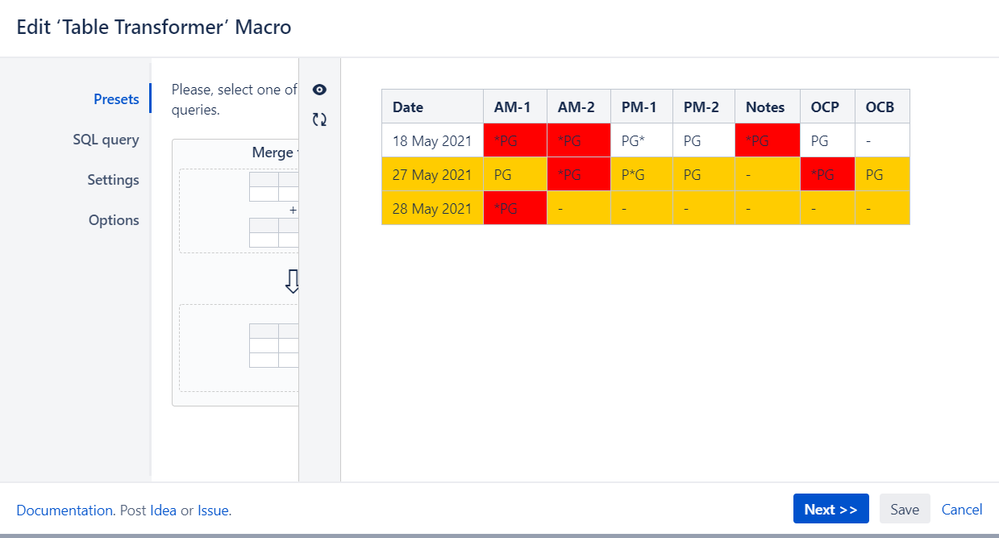As you can see, I've modified your conditions using the LIKE "*%" expression to find your "*SOME TEXT" cells and color them differently.

Hope this helps.

Paul Gwin likes this

This is great! thanks @Katerina Kovriga _Stiltsoft_
for the 2nd main query, im looking to do 2 sets of formatting.
The 1st is to color the bg based on week is current, but then I also want to separately mark the inner TEXT red if it starts with '*'. I thought about prepopulating the empty cells, but wasn't sure if there was a way to do it with null.

I will try that

-Paul

It is also possible to change the text color with a rather similar SQL, here is an example from our documentation.

I'll get back to my test instance tomorrow and provide you with a new query.

Hi @Paul Gwin ,

I've turned to our developers about your combined case related to the formatting both by background and text color and here is the result SQL query for your second Transformer:

SELECT

FORMATWIKI("{cell:bgColor=" + 'color' + "}" + FORMATDATE(T1.'Date') + "{cell}")
AS 'Date',

FORMATWIKI("{cell:bgColor=" + 'color' + "|textColor=" +
CASE WHEN T1.'AM-1' NOT LIKE "*%" THEN "BLACK"
ELSE "RED"
END
+ "}" + T1.'AM-1' + "{cell}")
AS 'AM-1',

FORMATWIKI("{cell:bgColor=" + 'color' + "|textColor=" +
CASE WHEN T1.'AM-2' NOT LIKE "*%" THEN "BLACK"
ELSE "RED"
END
+ "}" + T1.'AM-2' + "{cell}")
AS 'AM-2',

FORMATWIKI("{cell:bgColor=" + 'color' + "|textColor=" +
CASE WHEN T1.'PM-1' NOT LIKE "*%" THEN "BLACK"
ELSE "RED"
END
+ "}" + T1.'PM-1' + "{cell}")
AS 'PM-1',

FORMATWIKI("{cell:bgColor=" + 'color' + "|textColor=" +
CASE WHEN T1.'PM-2' NOT LIKE "*%" THEN "BLACK"
ELSE "RED"
END
+ "}" + T1.'PM-2' + "{cell}")
AS 'PM-2',

FORMATWIKI("{cell:bgColor=" + 'color' + "|textColor=" +
CASE WHEN T1.'Notes' NOT LIKE "*%" THEN "BLACK"
ELSE "RED"
END
+ "}" + T1.'Notes' + "{cell}")
AS 'Notes',

FORMATWIKI("{cell:bgColor=" + 'color' + "|textColor=" +
CASE WHEN T1.'OCP' NOT LIKE "*%" THEN "BLACK"
ELSE "RED"
END
+ "}" + T1.'OCP' + "{cell}")
AS 'OCP',

FORMATWIKI("{cell:bgColor=" + 'color' + "|textColor=" +
CASE WHEN T1.'OCB' NOT LIKE "*%" THEN "BLACK"
ELSE "RED"
END
+ "}" + T1.'OCB' + "{cell}")
AS 'OCB'

FROM (
SELECT *,
CASE WHEN DATEDIFF(day,"today",'Date') <= "7" AND DATEDIFF(day,"today",'Date') >= "0" THEN "#FFCC00"
ELSE "#FFFFFF" END AS 'color'
FROM T*) AS T1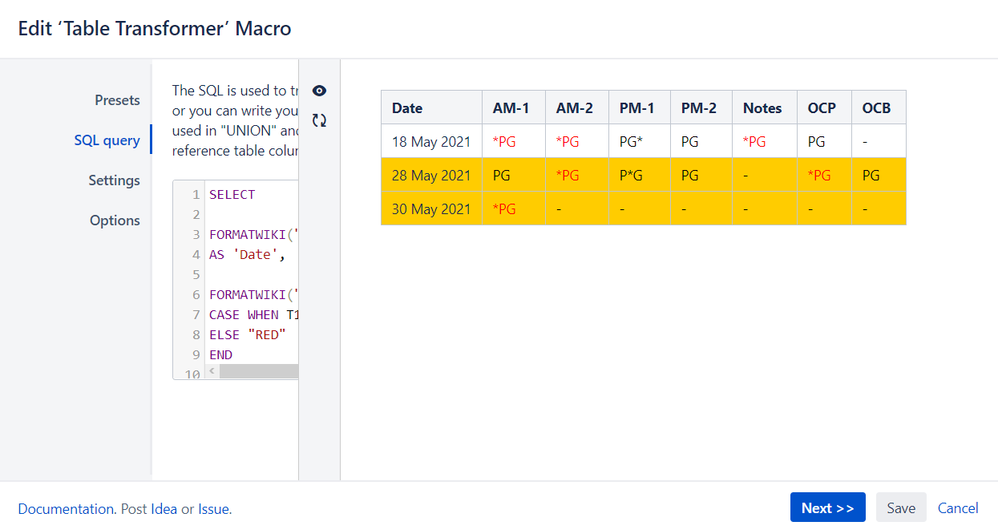The query is a rather complex one but it seems to fulfil your case.

@Katerina Kovriga _Stiltsoft_
thanks very much !!! that worked a treat ... i moded the date check slightly to slim it down, but im glad i can now cover the whole table from that one query ...

So thanks very much for you help and here is what I ended up with.

2 nested table transform macro's and the table in the inner table.

outer sql:
SELECT *,
CASE WHEN T1.'AM-1' IS NULL THEN "-"
ELSE T1.'AM-1'
END
AS 'AM-1',
CASE WHEN T1.'AM-2' IS NULL THEN "-"
ELSE T1.'AM-2'
END
AS 'AM-2',
CASE WHEN T1.'PM-1' IS NULL THEN "-"
ELSE T1.'PM-1'
END
AS 'PM-1',
CASE WHEN T1.'PM-2' IS NULL THEN "-"
ELSE T1.'PM-2'
END
AS 'PM-2',
CASE WHEN T1.'Notes' IS NULL THEN "-"
ELSE T1.'Notes'
END
AS 'Notes',
CASE WHEN T1.'OCP' IS NULL THEN "-"
ELSE T1.'OCP'
END
AS 'OCP',
CASE WHEN T1.'OCB' IS NULL THEN "-"
ELSE T1.'OCB'
END
AS 'OCB'
FROM T*

inner sql:

SELECT

FORMATWIKI("{cell:bgColor=" + 'color' + "}" + FORMATDATE(T1.'Date') + "{cell}")
AS 'Date',

FORMATWIKI("{cell:bgColor=" + 'color' + "|textColor=" +
CASE WHEN T1.'AM-1' NOT LIKE "*%" THEN "BLACK"
ELSE "RED"
END
+ "}" + T1.'AM-1' + "{cell}")
AS 'AM-1',

FORMATWIKI("{cell:bgColor=" + 'color' + "|textColor=" +
CASE WHEN T1.'AM-2' NOT LIKE "*%" THEN "BLACK"
ELSE "RED"
END
+ "}" + T1.'AM-2' + "{cell}")
AS 'AM-2',

FORMATWIKI("{cell:bgColor=" + 'color' + "|textColor=" +
CASE WHEN T1.'PM-1' NOT LIKE "*%" THEN "BLACK"
ELSE "RED"
END
+ "}" + T1.'PM-1' + "{cell}")
AS 'PM-1',

FORMATWIKI("{cell:bgColor=" + 'color' + "|textColor=" +
CASE WHEN T1.'PM-2' NOT LIKE "*%" THEN "BLACK"
ELSE "RED"
END
+ "}" + T1.'PM-2' + "{cell}")
AS 'PM-2',

FORMATWIKI("{cell:bgColor=" + 'color' + "|textColor=" +
CASE WHEN T1.'Notes' NOT LIKE "*%" THEN "BLACK"
ELSE "RED"
END
+ "}" + T1.'Notes' + "{cell}")
AS 'Notes',

FORMATWIKI("{cell:bgColor=" + 'color' + "|textColor=" +
CASE WHEN T1.'OCP' NOT LIKE "*%" THEN "BLACK"
ELSE "RED"
END
+ "}" + T1.'OCP' + "{cell}")
AS 'OCP',

FORMATWIKI("{cell:bgColor=" + 'color' + "|textColor=" +
CASE WHEN T1.'OCB' NOT LIKE "*%" THEN "BLACK"
ELSE "RED"
END
+ "}" + T1.'OCB' + "{cell}")
AS 'OCB'

FROM (
SELECT *,
CASE WHEN DATEDIFF(week,"today",'Date') == 0 THEN "#FFCC00"
ELSE "#FFFFFF" END AS 'color'
FROM T*) AS T1

Resulting table: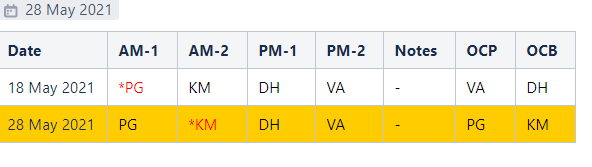Hi @Katerina Kovriga _Stiltsoft_ I came in this morning and my table is now not displaying any of the bg formatting?
Not sure how to debug this one ??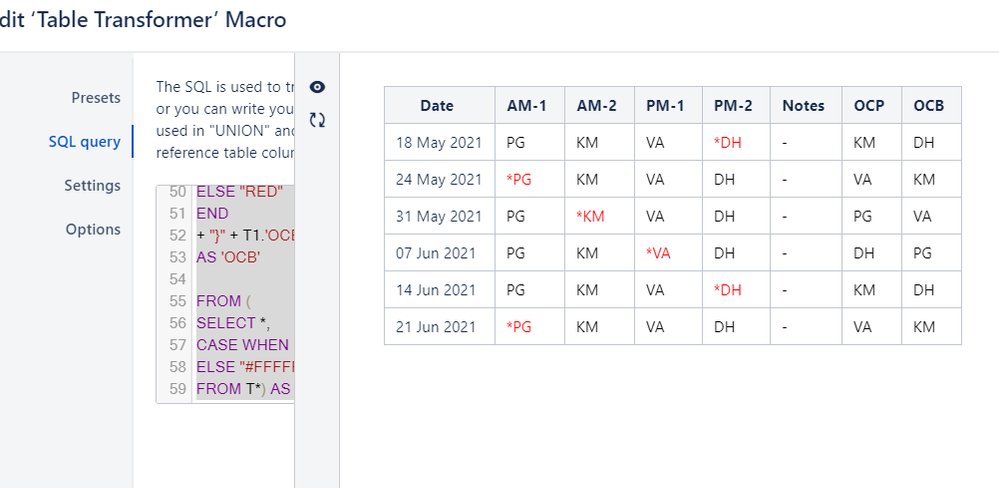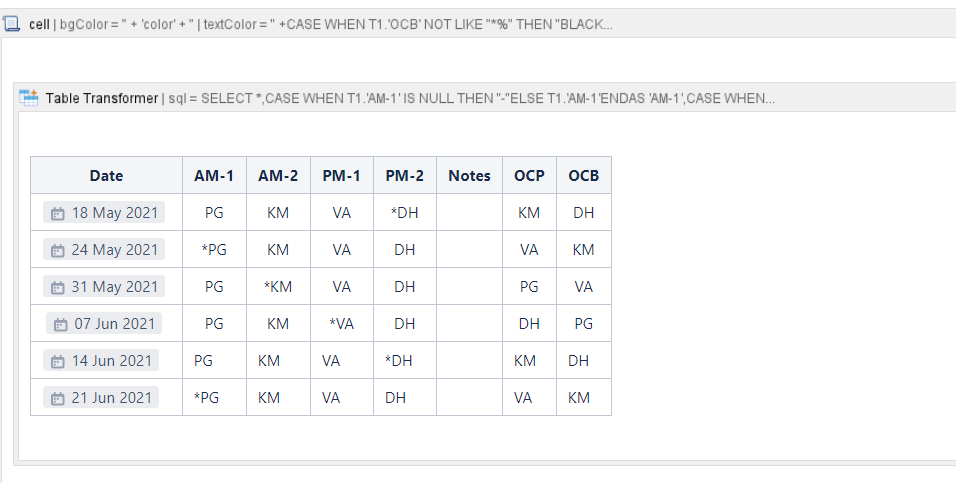Here is the full query from the table.  Even if i put the table into a singular table that doesn't format the empty cells with '-'

SELECT

FORMATWIKI("{cell:bgColor=" + 'color' + "}" + FORMATDATE(T1.'Date') + "{cell}")
AS 'Date',

FORMATWIKI("{cell:bgColor=" + 'color' + "|textColor=" +
CASE WHEN T1.'AM-1' NOT LIKE "*%" THEN "BLACK"
ELSE "RED"
END
+ "}" + T1.'AM-1' + "{cell}")
AS 'AM-1',

FORMATWIKI("{cell:bgColor=" + 'color' + "|textColor=" +
CASE WHEN T1.'AM-2' NOT LIKE "*%" THEN "BLACK"
ELSE "RED"
END
+ "}" + T1.'AM-2' + "{cell}")
AS 'AM-2',

FORMATWIKI("{cell:bgColor=" + 'color' + "|textColor=" +
CASE WHEN T1.'PM-1' NOT LIKE "*%" THEN "BLACK"
ELSE "RED"
END
+ "}" + T1.'PM-1' + "{cell}")
AS 'PM-1',

FORMATWIKI("{cell:bgColor=" + 'color' + "|textColor=" +
CASE WHEN T1.'PM-2' NOT LIKE "*%" THEN "BLACK"
ELSE "RED"
END
+ "}" + T1.'PM-2' + "{cell}")
AS 'PM-2',

FORMATWIKI("{cell:bgColor=" + 'color' + "|textColor=" +
CASE WHEN T1.'Notes' NOT LIKE "*%" THEN "BLACK"
ELSE "RED"
END
+ "}" + T1.'Notes' + "{cell}")
AS 'Notes',

FORMATWIKI("{cell:bgColor=" + 'color' + "|textColor=" +
CASE WHEN T1.'OCP' NOT LIKE "*%" THEN "BLACK"
ELSE "RED"
END
+ "}" + T1.'OCP' + "{cell}")
AS 'OCP',

FORMATWIKI("{cell:bgColor=" + 'color' + "|textColor=" +
CASE WHEN T1.'OCB' NOT LIKE "*%" THEN "BLACK"
ELSE "RED"
END
+ "}" + T1.'OCB' + "{cell}")
AS 'OCB'

FROM (
SELECT *,
CASE WHEN DATEDIFF(week,"today",'Date') == 0 THEN "#FFCC00"
ELSE "#FFFFFF" END AS 'color'
FROM T*) AS T1

The original query works just fine - I recommend to use it:

SELECT

FORMATWIKI("{cell:bgColor=" + 'color' + "}" + FORMATDATE(T1.'Date') + "{cell}")
AS 'Date',

FORMATWIKI("{cell:bgColor=" + 'color' + "|textColor=" +
CASE WHEN T1.'AM-1' NOT LIKE "*%" THEN "BLACK"
ELSE "RED"
END
+ "}" + T1.'AM-1' + "{cell}")
AS 'AM-1',

FORMATWIKI("{cell:bgColor=" + 'color' + "|textColor=" +
CASE WHEN T1.'AM-2' NOT LIKE "*%" THEN "BLACK"
ELSE "RED"
END
+ "}" + T1.'AM-2' + "{cell}")
AS 'AM-2',

FORMATWIKI("{cell:bgColor=" + 'color' + "|textColor=" +
CASE WHEN T1.'PM-1' NOT LIKE "*%" THEN "BLACK"
ELSE "RED"
END
+ "}" + T1.'PM-1' + "{cell}")
AS 'PM-1',

FORMATWIKI("{cell:bgColor=" + 'color' + "|textColor=" +
CASE WHEN T1.'PM-2' NOT LIKE "*%" THEN "BLACK"
ELSE "RED"
END
+ "}" + T1.'PM-2' + "{cell}")
AS 'PM-2',

FORMATWIKI("{cell:bgColor=" + 'color' + "|textColor=" +
CASE WHEN T1.'Notes' NOT LIKE "*%" THEN "BLACK"
ELSE "RED"
END
+ "}" + T1.'Notes' + "{cell}")
AS 'Notes',

FORMATWIKI("{cell:bgColor=" + 'color' + "|textColor=" +
CASE WHEN T1.'OCP' NOT LIKE "*%" THEN "BLACK"
ELSE "RED"
END
+ "}" + T1.'OCP' + "{cell}")
AS 'OCP',

FORMATWIKI("{cell:bgColor=" + 'color' + "|textColor=" +
CASE WHEN T1.'OCB' NOT LIKE "*%" THEN "BLACK"
ELSE "RED"
END
+ "}" + T1.'OCB' + "{cell}")
AS 'OCB'

FROM (
SELECT *,
CASE WHEN DATEDIFF(day,"today",'Date') <= "7" AND DATEDIFF(day,"today",'Date') >= "0" THEN "#FFCC00"
ELSE "#FFFFFF" END AS 'color'
FROM T*) AS T1

It seems that your condition "(week,"today",'Date') == 0" colors only today's date - enter the 01 Jun 2021 into your table and the line will turn yellow.

@Katerina Kovriga _Stiltsoft_  Hmm strange ..

I am trying to eval todays date as being part of the current week, which should equate to 0 in this case so it should match (i corrected == to =, but still same result).

Here is the test that im using:
DATEDIFF function https://www.w3schools.com/sql/func_sqlserver_datediff.asp

And function test:
https://www.w3schools.com/sql/trysqlserver.asp?filename=trysql_func_sqlserver_datediff
SELECT DATEDIFF(week, '31 May 21', '1 June 21') AS DateDiff;

@Paul Gwin Try this variant:

SELECT

FORMATWIKI("{cell:bgColor=" + 'color' + "}" + FORMATDATE(T1.'Date') + "{cell}")
AS 'Date',

FORMATWIKI("{cell:bgColor=" + 'color' + "|textColor=" +
CASE WHEN T1.'AM-1' NOT LIKE "*%" THEN "BLACK"
ELSE "RED"
END
+ "}" + T1.'AM-1' + "{cell}")
AS 'AM-1',

FORMATWIKI("{cell:bgColor=" + 'color' + "|textColor=" +
CASE WHEN T1.'AM-2' NOT LIKE "*%" THEN "BLACK"
ELSE "RED"
END
+ "}" + T1.'AM-2' + "{cell}")
AS 'AM-2',

FORMATWIKI("{cell:bgColor=" + 'color' + "|textColor=" +
CASE WHEN T1.'PM-1' NOT LIKE "*%" THEN "BLACK"
ELSE "RED"
END
+ "}" + T1.'PM-1' + "{cell}")
AS 'PM-1',

FORMATWIKI("{cell:bgColor=" + 'color' + "|textColor=" +
CASE WHEN T1.'PM-2' NOT LIKE "*%" THEN "BLACK"
ELSE "RED"
END
+ "}" + T1.'PM-2' + "{cell}")
AS 'PM-2',

FORMATWIKI("{cell:bgColor=" + 'color' + "|textColor=" +
CASE WHEN T1.'Notes' NOT LIKE "*%" THEN "BLACK"
ELSE "RED"
END
+ "}" + T1.'Notes' + "{cell}")
AS 'Notes',

FORMATWIKI("{cell:bgColor=" + 'color' + "|textColor=" +
CASE WHEN T1.'OCP' NOT LIKE "*%" THEN "BLACK"
ELSE "RED"
END
+ "}" + T1.'OCP' + "{cell}")
AS 'OCP',

FORMATWIKI("{cell:bgColor=" + 'color' + "|textColor=" +
CASE WHEN T1.'OCB' NOT LIKE "*%" THEN "BLACK"
ELSE "RED"
END
+ "}" + T1.'OCB' + "{cell}")
AS 'OCB'

FROM (
SELECT *,
CASE WHEN FLOOR(DATEDIFF(week,"today",'Date')) == 0 THEN "#FFCC00"
ELSE "#FFFFFF" END AS 'color'
FROM T*) AS T1

Here is the description of the FLOOR function: DATEDIFF returns non integer values (like the difference between the two dates is 0.4 of a full week) and you need to take the less round integer value.

Paul Gwin likes this

@Katerina Kovriga _Stiltsoft_  Thanks kindly !

FLOOR seems to work, but cant seem to debug that use of floor in any of my sql debuggers? I understand you explanation, just trying to run multiple tests on that usage. :)  thanks kindly for helping though!

The Table Transformer macro is based on the AlaSQL library, its functions may differ a little bit.

Paul Gwin likes this

You can try our app Excellentable to create conditional formats just the way you do them in Excel/Google sheets.
You can also import a .xlsx file with conditional formatting directly to Excellentable.

Here is an example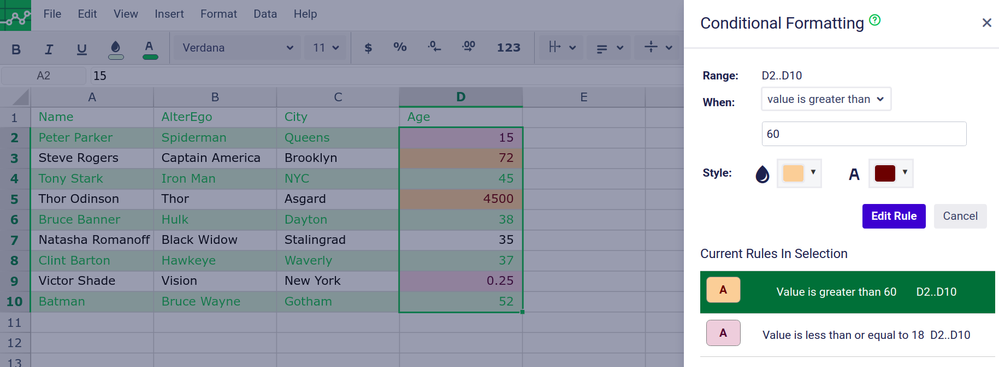Pansilu,

Welcome to the community.  The only way I know of to do this in Confluence Cloud is using an app like CelesteCS Conditions for Confluence.  On server you can write a user macro but in cloud I believe an app is the only option you have.

CLOUD
STANDARD
###### TAGS
Community showcase

### Confluence Mythbusters: Does Atlassian even use Confluence?

Hi, Confluence collaborators! As part of #Confluence-Collaboratory month, we’ve created a very special Mythsbusters segment, where we're dive into an interesting myth and uncover the truth behind i...

1,469 views 7 29

### Community Events

Connect with like-minded Atlassian users at free events near you!

Connect with like-minded Atlassian users at free events near you!

Unfortunately there are no Community Events near you at the moment.